# Loops and Branching

• 57k Accesses

Part of the Texts in Computational Science and Engineering book series (TCSE,volume 15)

## Abstract

Many computations are repetitive by nature and programming languages have certain loop structures to deal with this. One such loop structure is the for loop.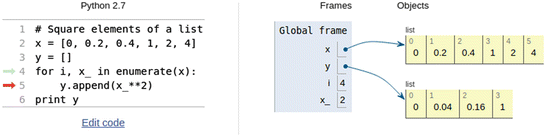## 3.1 The for Loop

Many computations are repetitive by nature and programming languages have certain loop structures to deal with this. One such loop structure is the for loop.

### 3.1.1 Example: Printing the 5 Times Table

Assume the task is to print out the 5 times table. Before having learned about loop structures in programming, most of us would first think of coding this like: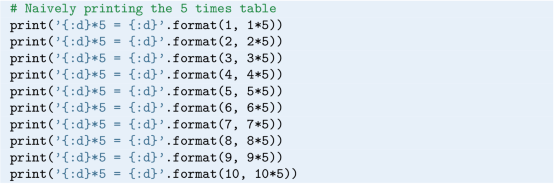When executed, the 10 results are printed quite nicely as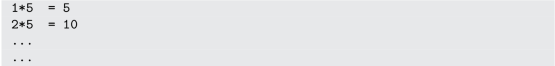With a for loop, however, the very same printout may be produced by just two (!) lines of code: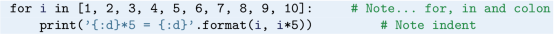With this construction, the loop variable i takes on each of the values 1 to 10, and for each value, the print function is called.

Since the numbers 1 to 10 appear in square brackets, they constitute a special structure called a list. The loop here would work equally well if the brackets had been dropped, but then the numbers would be a tuple:Both lists and tuples have certain properties, which we will come back to in Sect. 5.1.

### Loop Structure

There are different ways to write for loops, but herein, they are typically structured as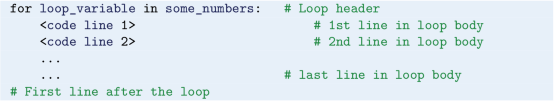where loop_variable runs through the numbersFootnote 1 given by some_numbers. In the very first line, called the for loop header, there are two reserved words, for and in. They are compulsory, as is the colon at the end. Also, the block of code lines inside a loop must be indented. These indented lines are referred to as the loop body. Once the indent is reversed, we are outside (and after) the loop (as commented with # First line after the loop, see code). One run-through of the loop body is called an iteration, i.e., in our example above with the 5 times table, the loop will do 10 iterations.

### Loop Variable

The name picked for the loop_variable is up to the programmer. In our times table example, the loop variable i appeared explicitly in the print command within the loop. Generally, however, the loop variable is not required to enter in any of the code lines within the loop, it is just available if you need it. This means that if we had, e.g., switched the print command inside our loop with print('Hello!') (i.e., so that i does not appear explicitly within the loop), i would still run through the numbers 1 to 10 as before, but Hello! would be printed 10 times instead.

The loop variable i takes on the values 1 to 10 in the order listed, and any order would be acceptable to Python. Thus, if we (for some reason) would like to reverse the order of the printouts, we could simply reverse the list of numbers, writing [10, 9, 8, 7, 6, 5, 4, 3, 2, 1] instead.

It should be noted that the loop variable is not restricted to run over integers. Our next example includes looping also over floating point values.

### Indentation and Nested Loops

In our simple times table example above, the print command inside the loop was indented 4 spaces, which is in accordance with the official style guide of Python.Footnote 2

Strictly speaking, the style guide recommends an indent of 4 spaces per indentation level. What this means, should become clear if we demonstrate how a for loop may appear within another for loop, i.e., if we show an arrangement with nested loops.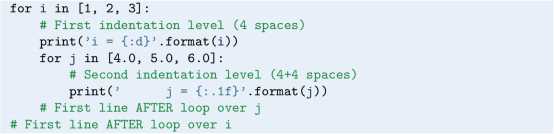The meaning of indentation levels should be clear from the comments (see code), and it is straight forward to use more nested loops than shown here (see, e.g., Exercise 5.7). Note that, together with the colon, indenting is part of the syntax also for other basic programming constructions in Python (e.g., in if-elif-else constructions and functions).

When executing the nested loop construction, we get this printout: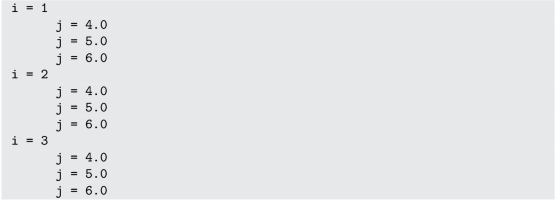From the printout, we may infer how the execution proceeds. For each value of i, the loop over j runs through all its values before i is updated (or the loop is terminated). To test your understanding of nested loops, you are recommended to do Exercise 5.1.

### Other for Loop Structures

In this book, you will occasionally meet for loops with a different structure, and as an example, take a look at this: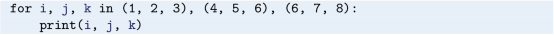Here, in the first iteration, the loop variables i, j and k will become 1, 2 and 3, respectively. In the second iteration, they will become 4, 5 and 6, respectively, and so on. Thus, the printout reads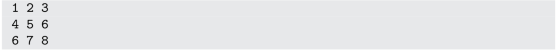As usual, each of i, j and k can be used in any desirable way within the loop.

### 3.1.3 Combining for Loop and Array

Often, loops are used in combination with arrays, so we should understand how that works. To reach this understanding, it is beneficial to do an example with just a small array.

Assume the case is to compute the average height of family members in a family of 5. We may choose to store all the heights in an array, which we then run through by use of a for loop to compute the average. The code (average_height.py) may look like this: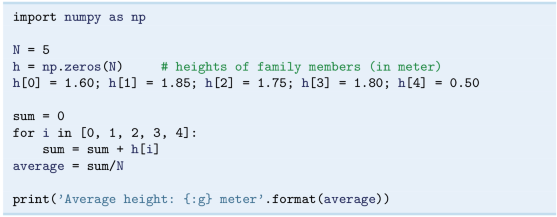When executed, the code gives 1.5 m as the average height, which compares favorably to a simple hand calculation. What happens here, is that we first sum upFootnote 3 all the heights being stored in the array h, before we divide by the number of family members N (i.e., just like we would do by hand). Observe how sum is initialized to 0 before entering the loop, and that with each iteration, a new height is added to sum. Note that the loop variable i takes on the integer index values of the array, which start with 0 and end with N − 1.

### Running Through Code “by Hand”

It is appropriate to stress that much understanding is often developed by first going through code “by hand”, i.e. just read the code while doing calculations by hand, before comparing these hand calculations to what the code produces when run (often some print commands must be inserted in the code to enable detailed comparison).

Thus, to make sure you understand the important details in average_height. py, you are encouraged to go through that code by hand right now. Also, in a copy, insert a print command in the loop, so that you can compare the output from that program to your own calculations, iteration by iteration.

### 3.1.4 Using the range Function

At this point, the observant reader might argue: “Well, this for loop seems handy, but what if the loop must do a really large number of iterations? If the loop variable is to run through hundreds of numbers, we must spend all day typing those numbers into the loop header”!

### An Example

This is where the built-in range function enters the picture. When called, the range function will provide integers according to the arguments given in the function call. For example, we could have used range in average_height.py by just changing the header from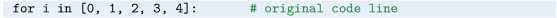to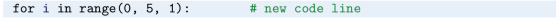Here, range( 0, 5, 1) is a function call, where the function range is told to provide the integers from 0 (inclusive) to 5 (exclusive!) in steps of 1. In this case, range( 0, 5, 1) will provide exactly those numbers that we had in the original code, i.e., the loop variable i will run through the same values (0, 1, 2, 3 and 4) as before, and program computations stay the same.

With a little interactive test, we may confirm that the range function provides the promised numbers. However, since what is returned from the range function is an object of type range, the number sequence is not explicitly available.Footnote 4 Converting the range object to a list, however, does the trick.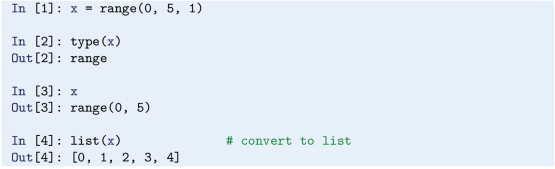### A General Call to range

With a header like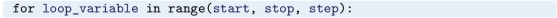and a step > 0, loop_variable will run through the numbers start, start + 1*step, start + 2*step, …., start + n*step, where start + n ∗ step < stop <= start + (n + 1) ∗ step. So, the final number is as close as we can get to the specified stop without equalling, or passing, it. For a negative step (step < 0, example given below), the same thing applies, meaning that the final number can not equal, or be more negative, than the argument stop. Note that an integer step different from 1 and − 1 is perfectly legal.

### Different Ways of Calling range

The function range is most often used in for loops to produce a required sequence of integers, but the function is not restricted to for loops only, of course. It may be called in different ways, e.g., utilizing default values. Some examples are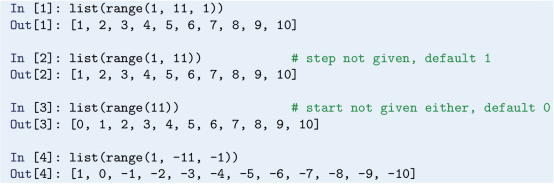When calling range, there is no argument specifying how many numbers should be produced, like we saw with the linspace function of numpy. With range, this is implied by the function arguments start, stop and step.

### Computer Memory Considerations

Note that range does not return all the requested numbers at once. Rather, the call to range will cause the numbers to be provided one by one during loop execution, giving one number per iteration. This way, simultaneous storing of all (loop variable) numbers in computer memory is avoided, which may be very important when there is a large number of loop iterations to make.

### 3.1.5 Using break and continue

It is possible to break out of a loop, i.e., to jump directly to the first code line after the loop, by use of a break statement. This might be desirable, for example, if a certain condition is met during loop execution, which makes the remaining iterations redundant.

With loops, it may also become relevant to skip any remaining statements of an ongoing iteration, and rather proceed directly with the next iteration. That is, contrary to the “loop stop” caused by a break statement, the loop continues to run after a continue statement, unless the end of the loop has been reached.

An example of how to use break and continue can be found in Sect. 5.2 (times_tables_4.py).

The break and continue statements may also be used in while loops, to be treated next.

## 3.2 The while Loop

The other basic loop construction in Python is the while loop, which runs as long as a condition is True. Let us move directly to an example, and explain what happens there, before we consider the loop more generally.

### 3.2.1 Example: Finding the Time of Flight

To demonstrate a while loop in action, we will make a minor modification of the case handled with ball_plot.py in Sect. 1.5. Now, we choose to find the time of flight for the ball.

### The Case

Assume the ball is thrown with a slightly lower initial velocity, say 4.5 ms−1, while everything else is kept unchanged. Since we still look at the first second of the flight, the heights at the end of the flight will then become negative. However, this only means that the ball has fallen below its initial starting position, i.e., the height where it left the hand, so there is nothing wrong with that. In an array y, we will then have a series of heights which towards the end of y become negative. As before, we will also have an array t with all the times for corresponding heights in y.

### The Program

In a program named ball_time.py, we may find the time of flight as the time when heights switch from positive to negative. The program could look like this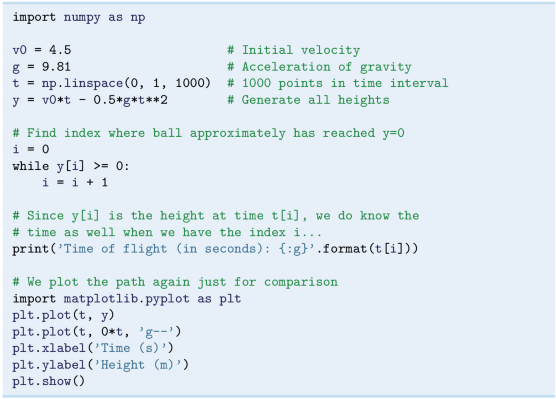The loop will run as long as the condition y[i] >= 0 evaluates to True. Note that the programmer introduced a variable by the name i, initialized it (i = 0) before the loop, and updated it (i = i + 1) in the loop. So, each time the condition y[i] >= 0 evaluates to True, i is explicitly increased by 1, allowing a check of successive elements in the array y.

When the condition y[i] >= 0 evaluates to False, program execution proceeds with the code lines after the loop. This means that, after skipping the comments, the time of flight is printed, followed by a plotting of the heights (to allow an easy check of the time of flight).

### Reporting the Answer

Remember that the height is computed at chosen points in time only, so, most likely, we do not have the time for when the height is exactly zero. Thus, reporting t[i] as the time of flight is an approximation. Another alternative, could be to report 0.5*( t[i-1] + t[i]) as the answer, reasoning that since y[i] is negative (which is why the loop terminated), t[i] must be too large.

### Running the Program

If you run this program, you get the printout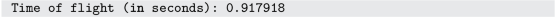and the plot seen in Fig. 3.1. The printed time of flight seems consistent with what we can read off from the plot.

### Loop Structure and Interpretation

The structure of a typical while loop may be put up as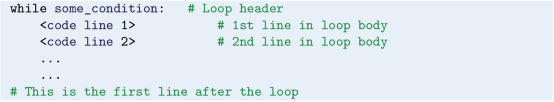The first line here is the while loop header. It contains the reserved word while and ends with a colon, both are compulsory. The indented lines that follow the header (i.e., <code line 1>, <code line 2>, etc.) constitute a block of statements, the loop body. Indentation is done as with for loops, i.e., 4 spaces by convention. In our example above with the ball, there was only a single line in the loop body (i.e., i = i + 1). As with for loops, one run-through of the loop body is referred to as an iteration. Once the indentation is reversed, the loop body has ended. Here, the first line after the loop is # This is the first line after the loop.

Between while and the colon, there is some_condition, which is a boolean expression that evaluates to either True or False. The boolean expression may be a compound expression with and, or, etc.

When a while loop is encountered by the Python interpreter, it evaluates some_condition the first time. If True, one iteration of the loop body is carried out. After this first iteration, some_condition is evaluated once again (meaning that program execution goes back up to the top of the loop). If True again, there is another iteration, and so on, just like we saw above with ball_time.py. Once some_condition evaluates to False, the loop is finished and execution continues with the first line after the loop. Note that if some_condition evaluates to False the very first time, the statements inside the loop will not be executed at all, and execution simply continues immediately with the first line after the loop.

Compared to a for loop, the programmer does not have to specify the number of iterations when coding a while loop. It simply runs until the boolean expression becomes False. Remember that if you want to use a variable analogously to the loop variable of a for loop, you have to explicitly update that variable inside the while loop (as we did with i in ball_time.py above). This differs from the automatic update of a loop variable in for loops.

Just as in for loops, there might be (arbitrarily) many code lines in a while loop. Also, nested loops work just like nested for loops. Having for loops inside while loops, and vice versa, is straight forward. Any for loop may also be implemented as a while loop, but while loops are more flexible, so not all of them can be expressed as a for loop.

### Infinite Loops

It is possible to have a while loop in which the condition never evaluates to False, meaning that program execution can not escape the loop! This is referred to as an infinite loop. Sometimes, infinite loops are just what you need, for example, in surveillance camera systems. More often, however, they are unintentional, and when learning to code, it is quite common to unintentionally end up with an infinite loop (just wait and see!). If you accidentally enter an infinite loop and the program just hangs “forever”, press Ctrl+c to stop the program.

To check that you have gained a basic understanding of the while loop construction, you are recommended to do Exercise 3.4.

## 3.3 Branching (if, elif and else)

Very often in life,Footnote 5 and in computer programs, the next action depends on the outcome of a question starting with “if”. This gives the possibility of branching into different types of action depending on some criterion.

As an introduction to branching, let us “build up” a little program that evaluates a water temperature provided by the program user.

### 3.3.1 Example: Judging the Water Temperature

Assume we want to write a program that helps us decide, based on water temperature alone (in degrees Celcius), whether we should go swimming or not.

### One if-test

As a start, we code our program simply as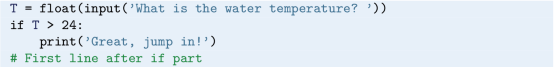Even if you have never seen an if test before, you are probably able to guess what will happen with this code. Python will first ask the user for the water temperature. Let us assume that 25 is entered, so that T becomes 25 through the assignment (note that, since the input returns a string, we convert it to a float before assigning to T). Next, the condition T >24 will evaluate to True, which implies that the print command gets executed and “Great, jump in!” appears on the screen.

To the contrary, if 24 (or lower) had been entered, the condition would have evaluated to False and the print command would not have been executed. Rather, execution would have proceeded directly to the line after the if part, i.e., to the line # First line after if part, and continued from there. This would mean, however, that our program would give us no response if we entered a water temperature of 24, or lower.

### Two if-tests

Immediately, we realize that this is not satisfactory, so (as a “first fix”) we extend our code with a second if test, as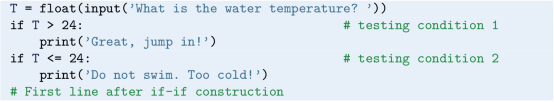This will work, at least in the way that we get a planned printout (“Do not swim. Too cold!”) also when the temperature is 24, or lower. However, something is not quite right here. If T is 24 (or lower), the first condition will evaluate to False, and Python will proceed immediately by also testing the second condition. However, this test is superfluous, since we know beforehand that it will evaluate to True! So, in this particular case, using two separate if tests is not suitable (generally, however, separate if tests may be just what you need. It all depends on the problem at hand).

### An if-else Construction

For our case, it is much better to use an else part, like this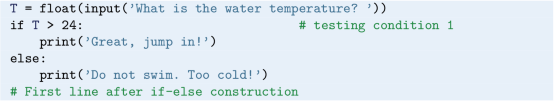When the first condition evaluates to False in this code, execution proceeds directly with the print command in the else part, with no extra testing!

To students with little programming experience, this may seem like a very small thing to shout about. However, in addition to avoiding an unnecessary test with the if-else alternative, it also corresponds better to the actual logic: If the first condition is false, then the other condition has to be true, and vice versa. No further checking is needed.

### An if-elif-else Construction

Considering our “advisor program”, we have to admit it is a bit crude, having only two categories. If the temperature is larger than 24 degrees, we are advised to swim, otherwise not. Some refinement seems to be the thing.

Let us say we allow some intermediate case, in which our program is less categoric for temperatures between 20 and 24 degrees, for example. There is a nice elif (short for else if) construction which then applies. Introducing that in our program (and saving it as swim_advisor.py), it reads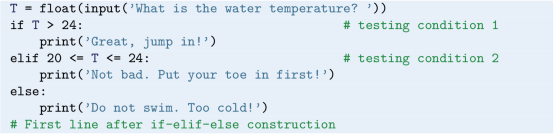You probably realize what will happen now. For temperatures above 24 and below 20, our “advisor” will respond just like in the previous version (i.e., the if-else version). However, for intermediate temperatures, the first condition will evaluate to False, which implies that the Python interpreter will continue with the elif line. Here, condition 2 will evaluate to True, which means that “Not bad. Put your toe in first!” will be printed. The else part is then skipped. As you might expect, more refinement would be straight forward to include by use of more elif parts.

### Programming as a Step-Wise Process

The reader should note that, besides demonstrating branching, the development of the previous program gave a (very) simple example of how code may be written by a step-wise approach. Starting out with the simplest version of the code, complexity is added step by step, before arriving at the final version. At each step, you make sure the code works as planned. Such a procedure is generally a good idea, and we will address it explicitly again, when we program a (slightly) more comprehensive case in Sect. 4.2.

### 3.3.2 The Characteristics of Branching

A more general form of an if-elif-else construction reads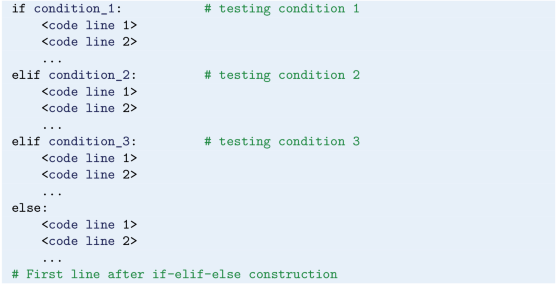Here we see an if part, two elif parts and an else part. Note the compulsory colon and indented code lines (a block of statements) in each case. As with loops, indents are conventionally 4 spaces. In such an arrangement, there may be “any” number of elif parts (also none) and the else part may, or may not, be present.

When interpreting an arrangement like this, Python starts checking the conditions, one after the other, from the top. If a condition (here, either condition_1, condition_2 or condition_3) evaluates to True, the corresponding code lines are executed, before proceeding directly to the first line after the whole arrangement (here, to the line # First line after if-elif-else construction). This means that any remaining tests, and the else part, are simply skipped! If none of the conditions evaluate to True, the else part (when present) is executed.

### 3.3.3 Example: Finding the Maximum Height

We have previously modified ball_plot.py from Sect. 1.5 to find the time of flight instead (see ball_time.py). Let us now change ball_plot.py in a slightly different way, so that the new program instead finds the maximum height achieved by the ball.

The solution illustrates a simple, and very common, search procedure, looping through an array by use of a for loop to find the maximum value. Our program ball_max_height.py reads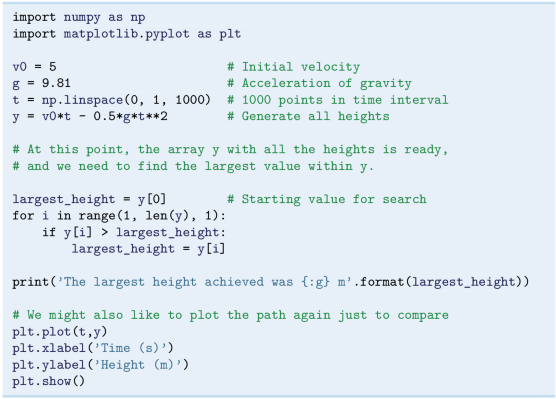We focus our attention on the new thing here, the search performed by the for loop. The value in y is used as a starting value for largest_height. The very first check then, tests whether y is larger than this height. If so, y is stored as the largest height. The for loop then updates i to 2, and continues to check y, and so on. Each time we find a larger number, we store it. When finished, largest_height will contain the largest number from the array y.

When you run the program, you get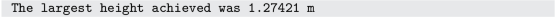which compares favorably to the plot that pops up (see Fig. 1.1).

The observant reader has already seen the similarity of finding the maximum height and finding the time of flight, as we addressed previously in Sect. 3.2.1. In fact, we could alternatively have solved the maximum height problem here by utilizing that y[i+1] >y[i] as the ball moves towards the top. Doing this, our search loop could have been written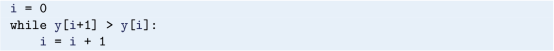When the condition y[i+1] >y[i] becomes False, we could report y[i+1] as our approximation of the maximum height, for example.

### Getting indices right

To implement the traversing of arrays with loops and indices, is often challenging to get right. You need to understand the start, stop and step length values for the loop variable, and also how the loop variable (possibly) enters expressions inside the loop. At the same time, however, it is something that programmers do often, so it is important to develop the right skills on these matters.

You are encouraged to test your understanding of the search procedure in ball_max_height.py by doing Exercise 3.9. That exercise will ask you to compare what you get “by hand” to printouts from the code. It is of fundamental importance to get this procedure as an established habit of yours, so do the exercise right now!

### 3.3.4 Example: Random Walk in Two Dimensions

We will now turn to an example which represents the core of so-called random walk algorithms. These are used in many branches of science and engineering, including such different fields as materials manufacturing and brain research.

The procedure we will consider, is to walk a series of equally sized steps, and for each of those steps, there should be the same probability of going to the north (N), east (E), south (S), or west (W). No other directions are legal. How can we implement such an action in a computer program?

To prepare our minds for the coding, it might be useful to first reflect upon how this could be done for real. One way, is to use a deck of cards, letting the four suits correspond to the four directions: clubs to N, diamonds to E, hearts to S, and spades to W, for instance. We draw a card, perform the corresponding move, and repeat the process a large number of times. The resulting path mimics, e.g., a typical path followed by a diffusing molecule.

In a computer program, we can not draw cards, but we can draw random numbers. So, we may use a loop to repeatedly draw a random number, and depending on the number, we update the coordinates of our location. There are many ways to draw random numbers and “translate” them into our four directions, and the technical details will typically depend on the programming language. However, our technique here is universal: we draw a random number from the interval [0, 1) and let [0, 0.25) correspond to N, [0.25, 0.5) to E, [0.5, 0.75) to S, and [0.75, 1) to W. We decide to simulate 1000 steps, each of length 1 (e.g., meter), starting from Origo in our coordinate system. To enable plotting our path, we use two arrays for storing the coordinate history, one for the x-coordinates and one for the corresponding y-coordinates.

The suggested code random_walk_2D.py then reads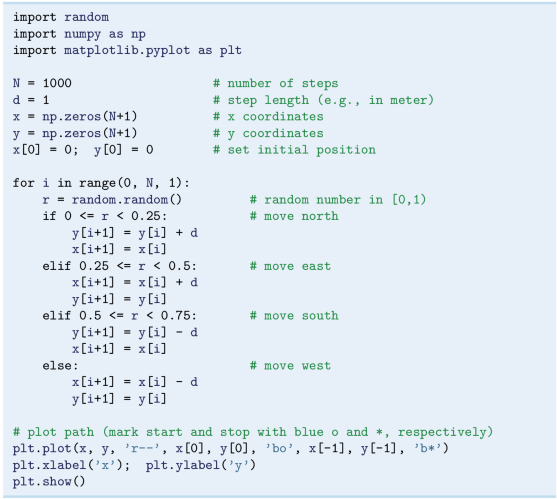Here, the initial position is explicitly set, even if x and y are known to be zero already. We do this, since the initial position is important, and by setting it explicitly, it is clearly not accidental what the starting position is. Note that if a step is taken in the x-direction, the y-coordinate is unchanged, and vice versa.

Executing the program produces the plot seen in Fig. 3.2, where the initial and final positions are marked in blue with a circle and a star, respectively. Remember that pseudo-random numbers are involved here, meaning that two consecutive runs will generally produce totally different paths.

## 3.4 Exercises

### Exercise 3.1: A for Loop with Errors

Assume some program has been written for the task of adding all integers i = 1, 2, …, 10 and printing the final result: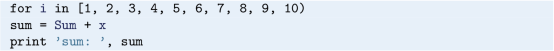1. a)

Identify the errors in the program by just reading the code.

2. b)

Write a new version of the program with errors corrected. Run this program and confirm that it gives the correct output.

Filename: for_loop_errors.py.

### Exercise 3.2: The range Function

Write a slightly different version of the program in Exercise 3.1. Now, the range function should be used in the for loop header, and only the even numbers from [2, 10] should be added. Also, the (only) statement within the loop should read sum = sum + i.

Filename: range_function.py.

### Exercise 3.3: A while Loop with Errors

Assume some program has been written for the task of adding all integers i = 1, 2, …, 10: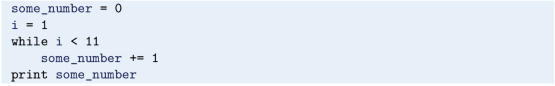1. a)

Identify the errors in the program by just reading the code.

2. b)

Write a new version of the program with errors corrected. Run this program and confirm that it gives the correct output.

Filename: while_loop_errors.py.

### Exercise 3.4: while Loop Instead of for Loop

Rewrite average_height.py from Sect. 3.1.3, using a while loop instead.

### Exercise 3.5: Compare Integers a and b

Explain briefly, in your own words, what the following program does.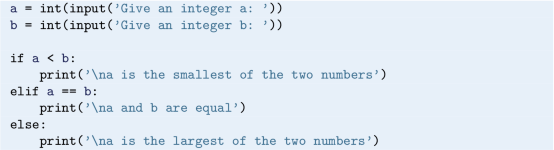Proceed by writing the program, and then run it a few times with different values for a and b to confirm that it works as intended. In particular, choose combinations for a and b so that all three branches of the if construction get tested.

Filename: compare_a_and_b.py.

Remarks

The program is not too robust, since it assumes the user types an integer as input (a real number gives trouble).

### Exercise 3.6: Area of Rectangle Versus Circle

Consider one circle and one rectangle. The circle has a radius r = 10.6. The rectangle has sides a and b, but only a is known from the outset. Let a = 1.3 and write a program that uses a while loop to find the largest possible integer b that gives a rectangle area smaller than, but as close as possible to, the area of the circle. Run the program and confirm that it gives the right answer (which is b = 271).

Filename: area_rectangle_vs_circle.py.

### Exercise 3.7: Frequency of Random Numbers

Write a program that takes a positive integer N as input and then draws N random integers from the interval [1, 6]. In the program, count how many of the numbers, M, that equal 6 and print out the fraction MN. Also, print all the random numbers to the screen so that you can check for yourself that the counting is correct. Run the program with a small value for N (e.g., N = 10) to confirm that it works as intended.

Hint

Use random.randint( 1,6) to draw a random integer between 1 and 6.

Filename: count_random_numbers.py.

Remarks

For large N, this program computes the probability MN of getting six eyes when throwing a dice.

### Exercise 3.8: Game 21

Consider some game where each participant draws a series of random integers evenly distributed between 0 and 10, with the aim of getting the sum as close as possible to 21, but not larger than 21. You are out of the game if the sum passes 21.

After each draw, you are told the number and your total sum, and are asked whether you want another draw or not. The one coming closest to 21 is the winner.

Implement this game in a program.

Hint

Use random.randint( 0,10) to draw random integers in [0, 10].

Filename: game_21.py.

### Exercise 3.9: Simple Search: Verification

Check your understanding of the search procedure in ball_max_height.py from Sect. 3.3.3 by comparing what you get “by hand” to printouts from the code. Work on a copy of ball_max_height.py. Comment out what you do not need, and use an array y of just 4 elements (or so). Fill that array with integers, so that you place a maximum value in a certain location. Then, run through that code by hand for every iteration of the loop, writing down the numbers in largest_height. Finally, place a print command in the loop, so that largest_height gets printed with every iteration. Run the program and compare to what you found by hand.

Filename: simple_search_verify.py.

### Exercise 3.10: Sort Array with Numbers

Write a script that uses the uniform function from the random module to generate an array of 6 random numbers between 0 and 10.

The program should then sort the array so that numbers appear in increasing order. Let the program make a formatted print of the array to screen both before and after sorting. Confirm that the array has been sorted correctly.

Filename: sort_numbers.py.

### Exercise 3.11: Compute π

Up through history, great minds have developed different computational schemes for the number π. We will here consider two such schemes, one by Leibniz (1646–1716), and one by Euler (1707–1783).

The scheme by Leibniz may be written

\displaystyle \begin{aligned} \pi = 8\sum_{k=0}^{\infty}\frac{1}{(4k + 1)(4k + 3)} , \end{aligned}

while one form of the Euler scheme may appear as

\displaystyle \begin{aligned} \pi = \sqrt[]{6\sum_{k=1}^{\infty}\frac{1}{k^2}} . \end{aligned}

If only the first N terms of each sum are used as an approximation to π, each modified scheme will have computed π with some error.

Write a program that takes N as input from the user, and plots the error development with both schemes as the number of iterations approaches N. Your program should also print out the final error achieved with both schemes, i.e. when the number of terms is N. Run the program with N = 100 and explain briefly what the graphs show.

Filename: compute_pi.py.

1. 1.

In Python, the loop variable does not have to be a number. For example, a header like for name in [‘John’, ‘Paul’, ‘George’, ‘Ringo’]: is fine, causing the loop variable to be a name. If you place print( name) inside the loop and run it, you get each of the names printed. In this book, however, our focus will be loops with numbers.

2. 2.
3. 3.

Note this way of using a loop to compute a sum, it is a standard technique in programming.

4. 4.

In Python 2, the range function returned the requested numbers as a list.

5. 5.

Some readers may perhaps be puzzled by this sentence, bringing in such a huge thing as life itself. The truth is, that this sentence is as deep as it appears. My dear co-author Hans Petter Langtangen wrote this sentence well into his cancer treatment. Hans Petter passed away on October 10th, 2016, a few months after the 1st edition of this book was published.

Authors

## Rights and permissions

Open Access This chapter is licensed under the terms of the Creative Commons Attribution 4.0 International License (http://creativecommons.org/licenses/by/4.0/), which permits use, sharing, adaptation, distribution and reproduction in any medium or format, as long as you give appropriate credit to the original author(s) and the source, provide a link to the Creative Commons license and indicate if changes were made.

The images or other third party material in this chapter are included in the chapter's Creative Commons license, unless indicated otherwise in a credit line to the material. If material is not included in the chapter's Creative Commons license and your intended use is not permitted by statutory regulation or exceeds the permitted use, you will need to obtain permission directly from the copyright holder.

Reprints and Permissions